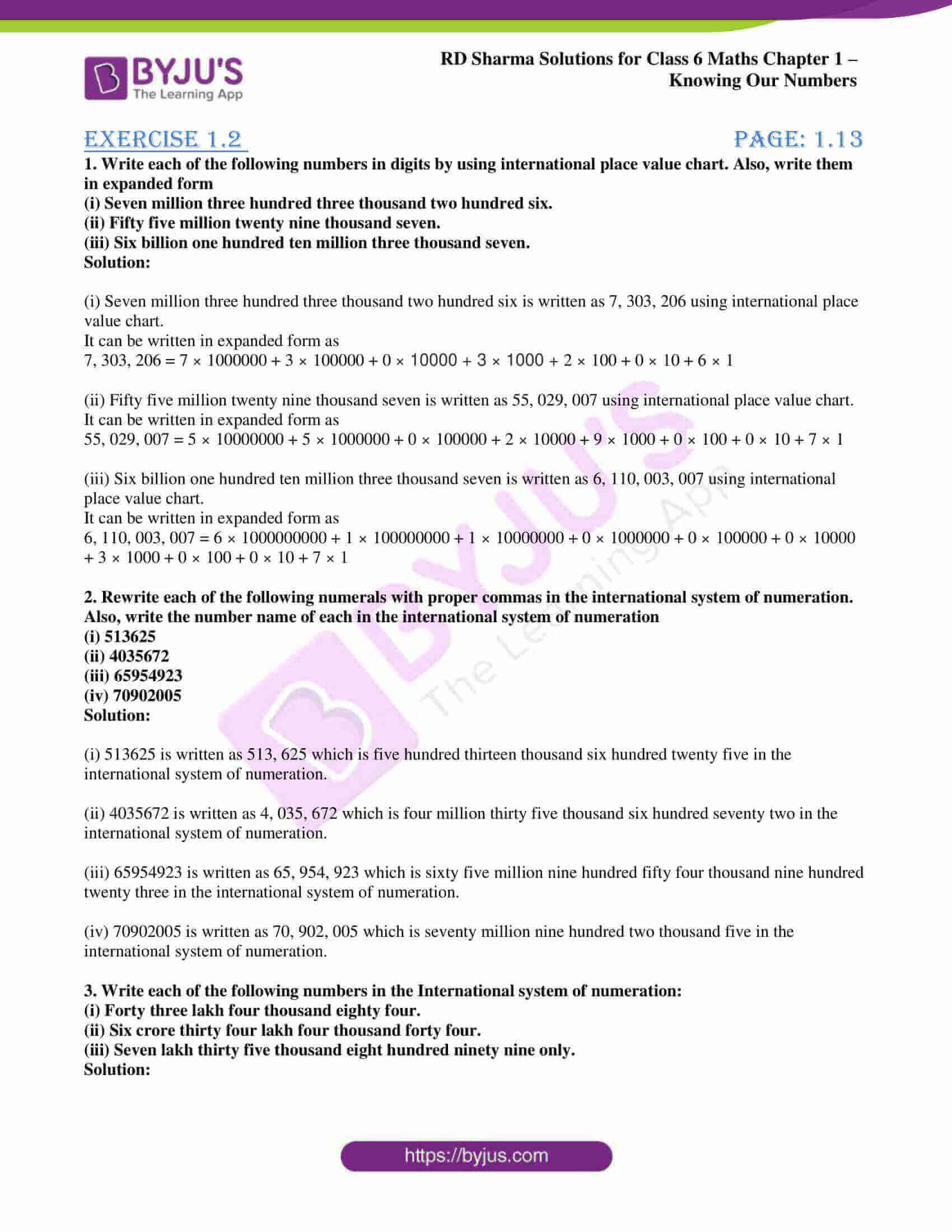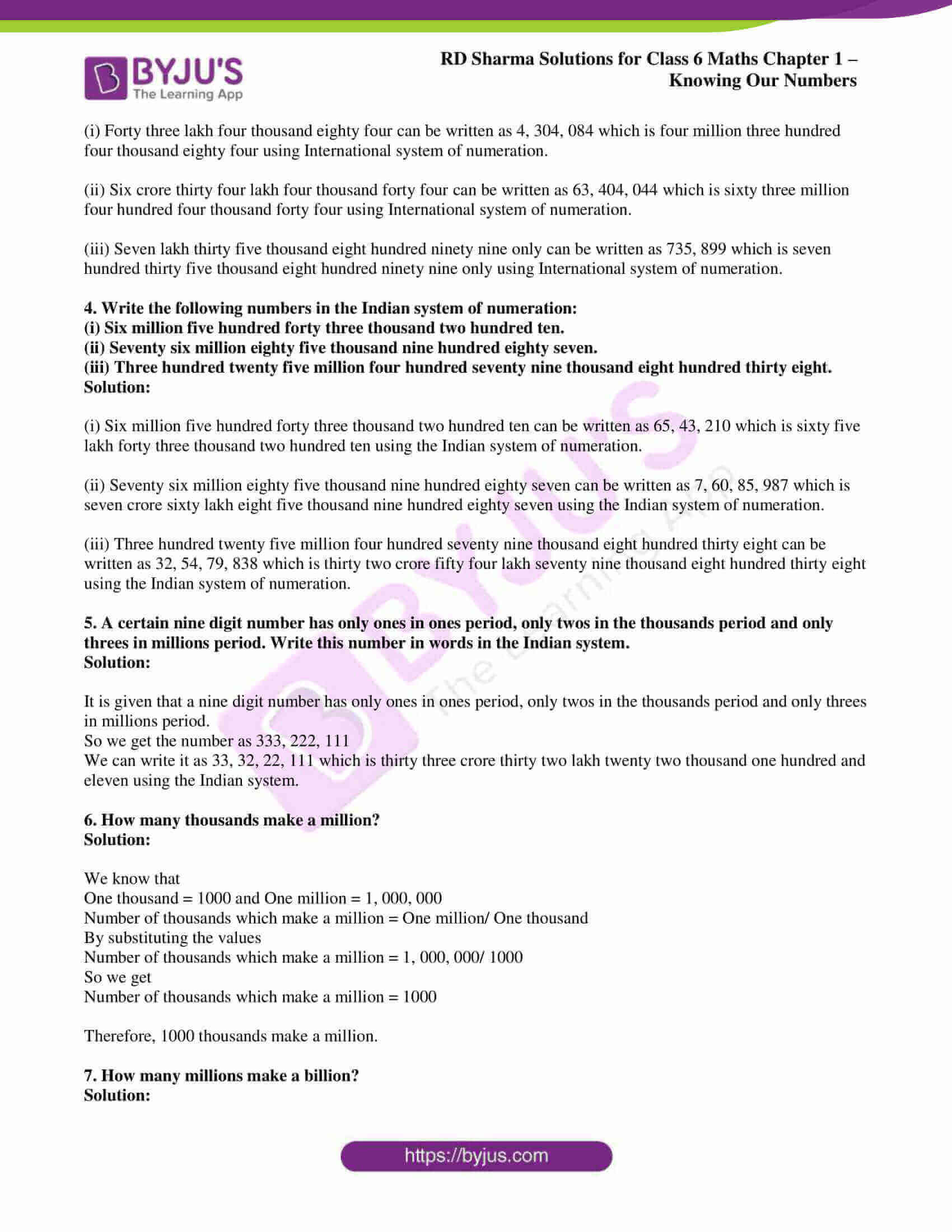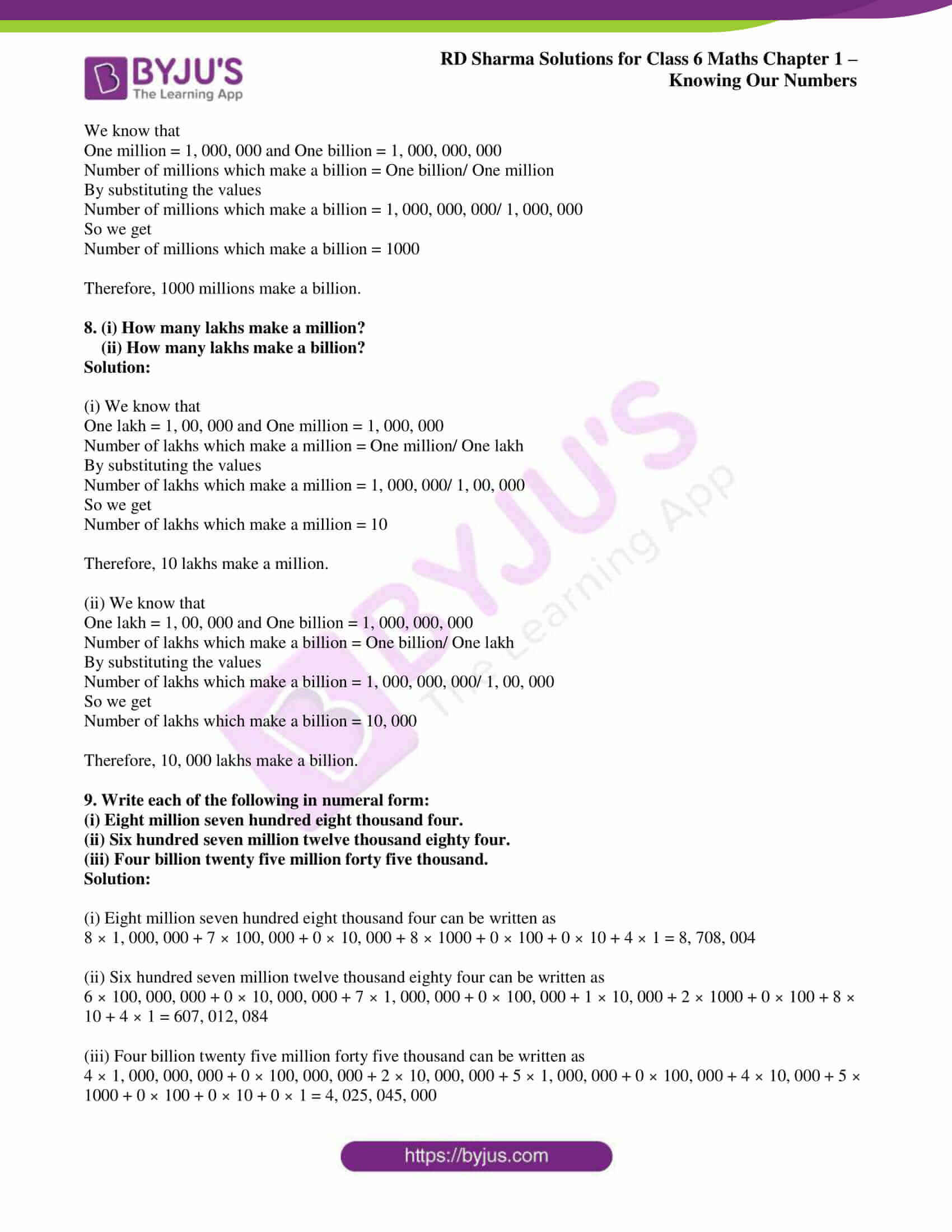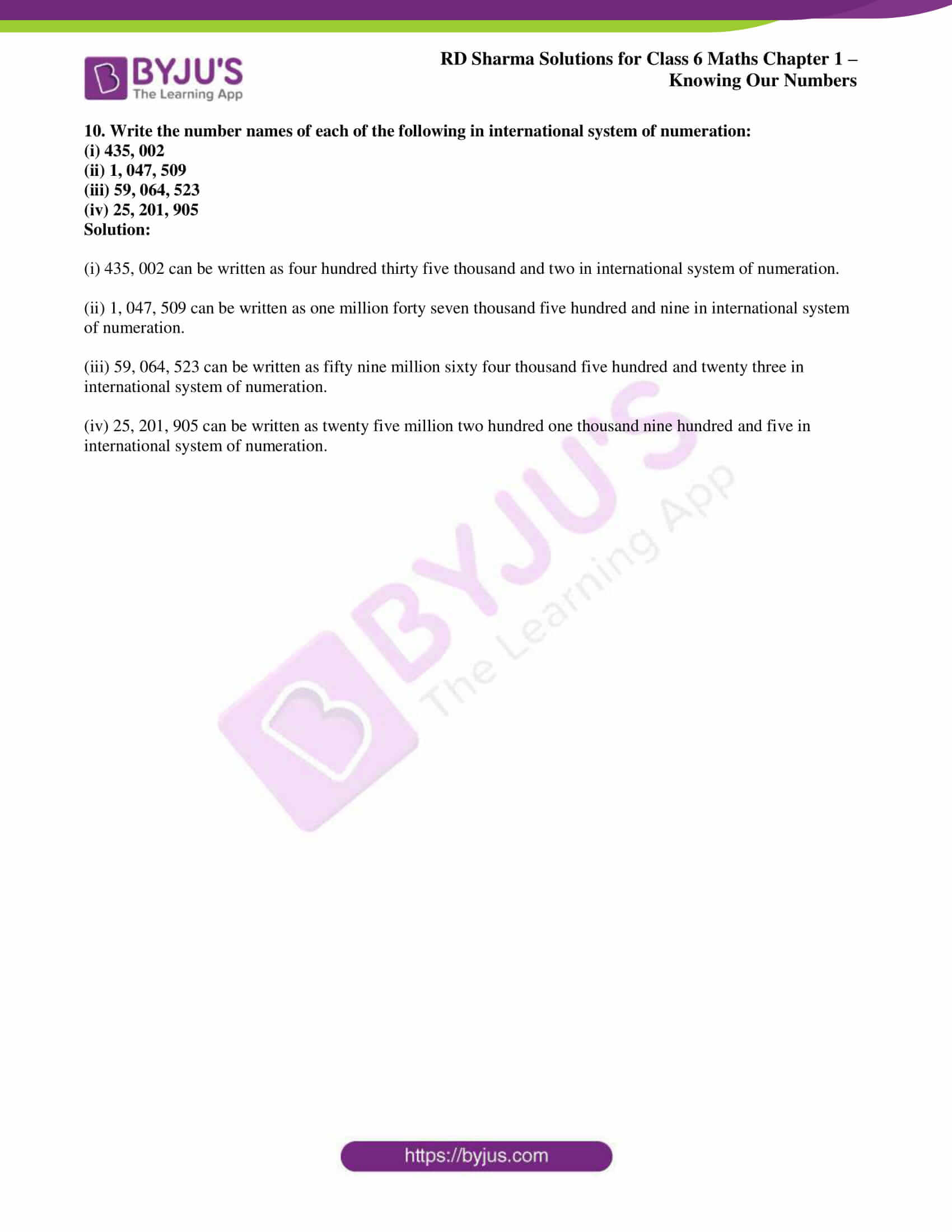# RD Sharma Solutions for Class 6 Maths Chapter 1: Knowing Our Numbers Exercise 1.2

## RD Sharma Solutions for Class 6 Maths Exercise 1.2 PDF

The exercise-wise solutions are depicted in an interactive manner to help students with their exam preparation. The expert faculty at BYJU’S provides students with PDF of solutions to solve the problems according to CBSE syllabus. The solutions for exercise wise problems are prepared after vast research is conducted on each topic. The step wise solutions prepared by subject experts help students to solve problems comfortably. Conceptual knowledge is crucial as some of the topics are continued in higher grades. RD Sharma Solutions for Class 6 Maths Chapter 1 Knowing Our Numbers Exercise 1.2 are provided here.

## RD Sharma Solutions for Class 6 Maths Chapter 1: Knowing Our Numbers Exercise 1.2 Download PDF### Access answers to Maths RD Sharma Solutions for Class 6 Chapter 1: Knowing Our Numbers Exercise 1.2

1. Write each of the following numbers in digits by using international place value chart. Also, write them in expanded form

(i) Seven million three hundred three thousand two hundred six.

(ii) Fifty five million twenty nine thousand seven.

(iii) Six billion one hundred ten million three thousand seven.

Solution:

(i) Seven million three hundred three thousand two hundred six is written as 7, 303, 206 using international place value chart.

It can be written in expanded form as

7, 303, 206 = 7 × 1000000 + 3 × 100000 + 0 × 10000 + 3 × 1000 + 2 × 100 + 0 × 10 + 6 × 1

(ii) Fifty five million twenty nine thousand seven is written as 55, 029, 007 using international place value chart.

It can be written in expanded form as

55, 029, 007 = 5 × 10000000 + 5 × 1000000 + 0 × 100000 + 2 × 10000 + 9 × 1000 + 0 × 100 + 0 × 10 + 7 × 1

(iii) Six billion one hundred ten million three thousand seven is written as 6, 110, 003, 007 using international place value chart.

It can be written in expanded form as

6, 110, 003, 007 = 6 × 1000000000 + 1 × 100000000 + 1 × 10000000 + 0 × 1000000 + 0 × 100000 + 0 × 10000 + 3 × 1000 + 0 × 100 + 0 × 10 + 7 × 1

2. Rewrite each of the following numerals with proper commas in the international system of numeration. Also, write the number name of each in the international system of numeration

(i) 513625

(ii) 4035672

(iii) 65954923

(iv) 70902005

Solution:

(i) 513625 is written as 513, 625 which is five hundred thirteen thousand six hundred twenty five in the international system of numeration.

(ii) 4035672 is written as 4, 035, 672 which is four million thirty five thousand six hundred seventy two in the international system of numeration.

(iii) 65954923 is written as 65, 954, 923 which is sixty five million nine hundred fifty four thousand nine hundred twenty three in the international system of numeration.

(iv) 70902005 is written as 70, 902, 005 which is seventy million nine hundred two thousand five in the international system of numeration.

3. Write each of the following numbers in the International system of numeration:

(i) Forty three lakh four thousand eighty four.

(ii) Six crore thirty four lakh four thousand forty four.

(iii) Seven lakh thirty five thousand eight hundred ninety nine only.

Solution:

(i) Forty three lakh four thousand eighty four can be written as 4, 304, 084 which is four million three hundred four thousand eighty four using International system of numeration.

(ii) Six crore thirty four lakh four thousand forty four can be written as 63, 404, 044 which is sixty three million four hundred four thousand forty four using International system of numeration.

(iii) Seven lakh thirty five thousand eight hundred ninety nine only can be written as 735, 899 which is seven hundred thirty five thousand eight hundred ninety nine only using International system of numeration.

4. Write the following numbers in the Indian system of numeration:

(i) Six million five hundred forty three thousand two hundred ten.

(ii) Seventy six million eighty five thousand nine hundred eighty seven.

(iii) Three hundred twenty five million four hundred seventy nine thousand eight hundred thirty eight.

Solution:

(i) Six million five hundred forty three thousand two hundred ten can be written as 65, 43, 210 which is sixty five lakh forty three thousand two hundred ten using the Indian system of numeration.

(ii) Seventy six million eighty five thousand nine hundred eighty seven can be written as 7, 60, 85, 987 which is seven crore sixty lakh eight five thousand nine hundred eighty seven using the Indian system of numeration.

(iii) Three hundred twenty five million four hundred seventy nine thousand eight hundred thirty eight can be written as 32, 54, 79, 838 which is thirty two crore fifty four lakh seventy nine thousand eight hundred thirty eight using the Indian system of numeration.

5. A certain nine digit number has only ones in ones period, only twos in the thousands period and only threes in millions period. Write this number in words in the Indian system.

Solution:

It is given that a nine digit number has only ones in ones period, only twos in the thousands period and only threes in millions period.

So we get the number as 333, 222, 111

We can write it as 33, 32, 22, 111 which is thirty three crore thirty two lakh twenty two thousand one hundred and eleven using the Indian system.

6. How many thousands make a million?

Solution:

We know that

One thousand = 1000 and One million = 1, 000, 000

Number of thousands which make a million = One million/ One thousand

By substituting the values

Number of thousands which make a million = 1, 000, 000/ 1000

So we get

Number of thousands which make a million = 1000

Therefore, 1000 thousands make a million.

7. How many millions make a billion?

Solution:

We know that

One million = 1, 000, 000 and One billion = 1, 000, 000, 000

Number of millions which make a billion = One billion/ One million

By substituting the values

Number of millions which make a billion = 1, 000, 000, 000/ 1, 000, 000

So we get

Number of millions which make a billion = 1000

Therefore, 1000 millions make a billion.

8. (i) How many lakhs make a million?

(ii) How many lakhs make a billion?

Solution:

(i) We know that

One lakh = 1, 00, 000 and One million = 1, 000, 000

Number of lakhs which make a million = One million/ One lakh

By substituting the values

Number of lakhs which make a million = 1, 000, 000/ 1, 00, 000

So we get

Number of lakhs which make a million = 10

Therefore, 10 lakhs make a million.

(ii) We know that

One lakh = 1, 00, 000 and One billion = 1, 000, 000, 000

Number of lakhs which make a billion = One billion/ One lakh

By substituting the values

Number of lakhs which make a billion = 1, 000, 000, 000/ 1, 00, 000

So we get

Number of lakhs which make a billion = 10, 000

Therefore, 10, 000 lakhs make a billion.

9. Write each of the following in numeral form:

(i) Eight million seven hundred eight thousand four.

(ii) Six hundred seven million twelve thousand eighty four.

(iii) Four billion twenty five million forty five thousand.

Solution:

(i) Eight million seven hundred eight thousand four can be written as

8 × 1, 000, 000 + 7 × 100, 000 + 0 × 10, 000 + 8 × 1000 + 0 × 100 + 0 × 10 + 4 × 1 = 8, 708, 004

(ii) Six hundred seven million twelve thousand eighty four can be written as

6 × 100, 000, 000 + 0 × 10, 000, 000 + 7 × 1, 000, 000 + 0 × 100, 000 + 1 × 10, 000 + 2 × 1000 + 0 × 100 + 8 × 10 + 4 × 1 = 607, 012, 084

(iii) Four billion twenty five million forty five thousand can be written as

4 × 1, 000, 000, 000 + 0 × 100, 000, 000 + 2 × 10, 000, 000 + 5 × 1, 000, 000 + 0 × 100, 000 + 4 × 10, 000 + 5 × 1000 + 0 × 100 + 0 × 10 + 0 × 1 = 4, 025, 045, 000

10. Write the number names of each of the following in international system of numeration:

(i) 435, 002

(ii) 1, 047, 509

(iii) 59, 064, 523

(iv) 25, 201, 905

Solution:

(i) 435, 002 can be written as four hundred thirty five thousand and two in international system of numeration.

(ii) 1, 047, 509 can be written as one million forty seven thousand five hundred and nine in international system of numeration.

(iii) 59, 064, 523 can be written as fifty nine million sixty four thousand five hundred and twenty three in international system of numeration.

(iv) 25, 201, 905 can be written as twenty five million two hundred one thousand nine hundred and five in international system of numeration.

### Key features of RD Sharma Solutions for Class 6 Maths Chapter 1: Knowing Our Numbers Exercise 1.2

RD Sharma Solutions Class 6 Maths Chapter 1 Knowing Our Numbers Exercise 1.2 explains the International System and Indian System of Numeration along with exercise wise solutions for each.

• PDF of exercise wise solutions prepared by subject experts are easily accessible by the students.
• Self analysis is improved by solving huge number of examples and exercise wise problems of RD Sharma textbook.
• Time management skills of students are increased with the help of RD Sharma Solutions for Class 6.
• The solutions are designed based on the latest CBSE guidelines and exam pattern.